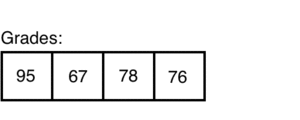# ArrayList Methods

Arrays are amazing tools! Unfortunately, they lack flexibility and useful methods. That's where ArrayList comes into play. An ArrayList is similar to an Array, except it is resizable and has more functionality.

### ArrayLists

You can think of an ArrayList as a container that will resize as we add and remove objects from it.### Creating ArrayLists

They way we create an ArrayList varies from the way we create an Array. To create an ArrayList we use: `ArrayList<type> variableName = new ArrayList<type>();`. It is also import to know ArrayLists can't store primitive types, so we must use `Integer` for ints and `Double` for doubles

Lets say we want to create an ArrayList that holds the grades for a class. Here is an example of what that code will look like:

``````// Import the ArrayList
import java.util.*;

public class ArrayListExample extends ConsoleProgram
{
public void run()
{
// Create the ArrayList named `classGrades` and set its type to `Integer`

}
}
``````

Now we want to create a list of students in a classroom. We can use the ArrayList for this as well:

``````// Import the ArrayList
import java.util.*;

public class ArrayListExample extends ConsoleProgram
{
public void run()
{
// Create the ArrayList named 'students' and set its type to 'String'
ArrayList<String> students = new ArrayList<String>();

// Add the students to our ArrayList
}
}
``````

### ArrayList Methods

After creating and populating your ArrayList you can use multiple methods with it.

To add a value to an ArrayList you use `list.add(elem);`. Here is an example using our `classGrades` and `students` ArrayLists:

``````classGrades.add(100);
``````
##### Adding at a Specific Index

ArrayLists also allow us to add an item at a specific index using `list.add(index, elem);`. Here is an example using our `classGrades` and `students` ArrayLists:

``````classGrades.add(1, 29); // Adds '29' to index 1, and shifts everything right
students.add(2, "Kyle"); // Adds 'Kyle' to index 2, and shifts everything right
``````
##### Getting a Value

To get a value from your ArrayList you use `list.get(index);`. Here is an example using our `classGrades` and `students` ArrayLists:

``````int grade = classGrades.get(2); // Will return `80`
String student = students.get(1); // Will return `Wezley`
``````
##### Setting a Value

With ArrayLists we can set a specific index's value using `list.set(index, value);`. Here is an example using our `classGrades` and `students` ArrayLists:

``````classGrades.set(1, 90); // Will change the value at index 1 to `90`
students.set(0, "Ryan"); // Will change the value at index 0 to `Ryan`
``````
##### Getting Size of an ArrayList

We can also access the length or size of a specific ArrayList using `list.size();`. Here is an example using our `classGrades` and `students` ArrayLists:

``````int gradeSize = classGrades.size(); // Will return 4
int classSize = students.size(); // Will return 3
``````
##### Removing From ArrayList

Finally, we can remove a specific item from our ArrayList using `list.remove(index);`. Here is an example using our `classGrades` and `students` ArrayLists:

``````classGrades.remove(1); // Removes `50` from `classGrades`
students.remove(0); // Removes `Stephen` from `students`
``````

### Iterating Over ArrayLists

As with traditional Arrays, we can iterate over ArrayLists.

We can use a regular for loop to iterate over our ArrayList like:

``````// Loops through `classGrades`
for(int i = 0; i < classGrades.size(); i++)
{
// Prints out our class grades
}
``````
``````// Loops through `students`
for(int i = 0; i < students.size(); i++)
{
// Prints out our students
String name = students.get(i);
System.out.println(name);
}
``````

We also have the option of using a For Each loop to iterate over our ArrayList like:

``````// Loops through `classGrades`
{
// Prints out the class grades.
``````// Loops through `students`